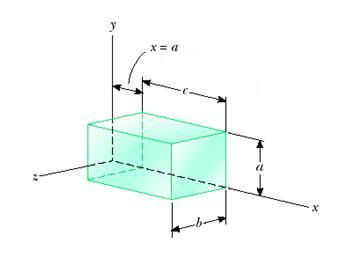# Electric flux through cube with nonuniform electric field

## Homework Statement

A closed surface with dimensions a=b=0.400m and c=0.600m is located as shown in the figure (be aware that the distance 'a' in the figure indicates that the rectangular box sits distance "a" from the y-z plane). The electric field throughout the region is nonuniform and given by E=(1.50-2.00x^2)i + (y)j + (1.00-z^3)k.

A. find the flux through each side (including units and signs)

B. find the total charge enclosed in the box.## Homework Equations

flux=EA
=integral of E.dA

## The Attempt at a Solution

for A)

now for the left and right sides i know x doesnt change, so that should just be E*A in the i direction, but y and z change so they should be integral of E.dA, and the total flux through that side would be the sum of those 3. but how do you solve an integration of y.dA? would you just evaluate (y^2)/2 from .4 to 0? if this is correct i can figure out the rest, but i dont think you can integrate y.dA, because you need dy right?

the top and bottom sides y doesnt change, so that would just be EA for the j direction, and for the front and back z doesnt change so it would be EA in the k. but the integrations are killing me....

B) since there is no enclosed charge it is 0 right?